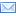#Difference Between Similar Terms and Objects

# Differences Between Rectangle and Trapezoid

Rectangle vs Trapezoid

Rectangles and trapezoids are both four-sided figures.

Rectangle
Any quadrilateral which is formed by right angles on four sides is termed as a rectangle. If a rectangle is not square, “oblong” is the term used. “Rectangle” as a term comes from “rectiangulus,” a Latin word, being a combination of “rectus” and “angulus” meaning “right” and “angle” respectively. A so-called crossed rectangle is the self-intersecting quadrilateral that consists of two opposite sides along with two diagonals.

Rectangles can generally be defined as a quadrilateral which has an axis of symmetry running through each pair on opposite sides. This definition of rectangle includes both crossed and right angle rectangles with each having an axis of symmetry equidistant and parallel from each pair on the opposite sides and another perpendicular axis bisector of the sides. However, in the case of a crossed rectangle, the first axis cannot be regarded as the axis of symmetry of either side which it bisects. A square is the special case of rectangle where all sides are equal. A parallelogram is also a special case of a rectangle without the restriction of angles being at 90 degrees each.

Properties of Rectangle:
General properties of rectangles are:

The diagonals are congruent.
The diagonals bisect each other.
Opposite sides are parallel and congruent.

Trapezoid
Trapezoid (called a trapezium outside America) is widely defined as a quadrilateral having at least one pair of parallel sides. The use of this definition is consistent in higher mathematics such as calculus. Thus, a parallelogram, rectangle, square, and rhombus are the special types of trapezoids. Some authors define it as having two pairs of parallel sides, but it is not a widely accepted concept.

Properties of a Trapezoid:
Assuming that trapezoid is a quadrilateral having one pair of opposite sides parallel, general properties of a trapezoid are:

Area is bisected by the line joining the midpoints of parallel sides.
If the trapezoid is divided into four triangles by joining the diagonals, then the areas of the triangles formed on the non-parallel sides are equal, and the product of these two triangular areas is equal to the product of the remaining two triangular areas.
The median is parallel to both bases.
Median length equals half the sum of the base lengths.

Summary:

1.Rectangles have four right angles while trapezoids do not.
2.Opposite sides of a rectangle are parallel and equal while in the case of a trapezoid the opposite sides of at least one pair are parallel.
3.Diagonals of rectangles must bisect each other while in the case of trapezoids that is not necessary.

Latest posts by Nimisha Kaushik (see all)

### Search DifferenceBetween.net :

Custom Search

Help us improve. Rate this post!Loading...Email This Post : If you like this article or our site. Please spread the word. Share it with your friends/family.

### 1 Comment

1. I will have to get a new one and it was so nice of you but have my great pleasure show

Please note: comment moderation is enabled and may delay your comment. There is no need to resubmit your comment.

Articles on DifferenceBetween.net are general information, and are not intended to substitute for professional advice. The information is "AS IS", "WITH ALL FAULTS". User assumes all risk of use, damage, or injury. You agree that we have no liability for any damages.

See more about :